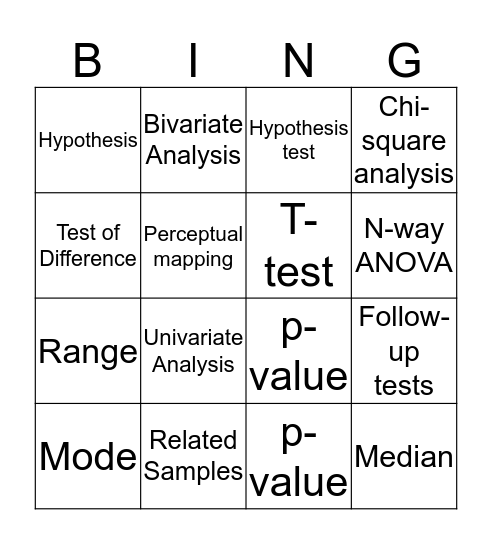# Marketing Research BingoThis bingo card has a free space and 44 words: Descriptive Analysis, Mode, Median, Mean, Bivariate Analysis, Multivariate Analysis, Hypothesis test, Hypothesis, Test of Difference, Cross-Tabulations, Chi-square analysis, Related Samples, Descriptive Analysis, Mode, Median, Descriptive Analysis, Mode, Median, Mean, Univariate Analysis, Bivariate Analysis, Multivariate Analysis, Hypothesis test, Hypothesis, Significance level, p-value, Test of Difference, Cross-Tabulations, Chi-square analysis, Independent samples, Related Samples, Independent Sample T-test, Paired Sample T-test, Analysis of Variance(ANOVA), Follow-up tests, One-way ANOVA, F-test, Interaction effect, N-way ANOVA, Perceptual mapping, Range, Standard Deviation, T-test and Variance.

⚠ This card has duplicate items: Descriptive Analysis (3), Mode (3), Median (3), Mean (2), Bivariate Analysis (2), Multivariate Analysis (2), Hypothesis test (2), Hypothesis (2), Test of Difference (2), Cross-Tabulations (2), Chi-square analysis (2), Related Samples (2)

## Play Online